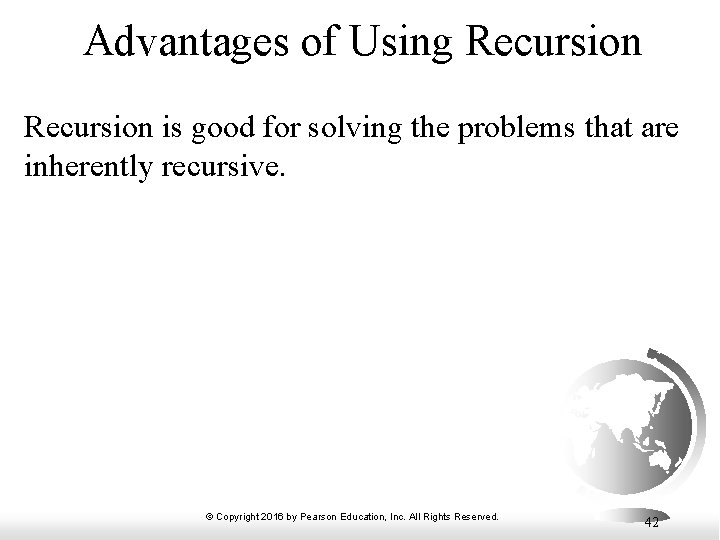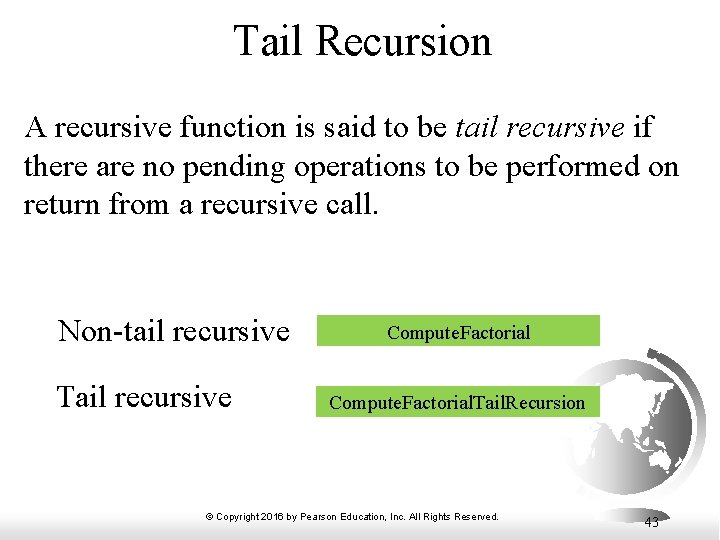# Chapter 17 Recursion Copyright 2016 by Pearson Education

• Slides: 43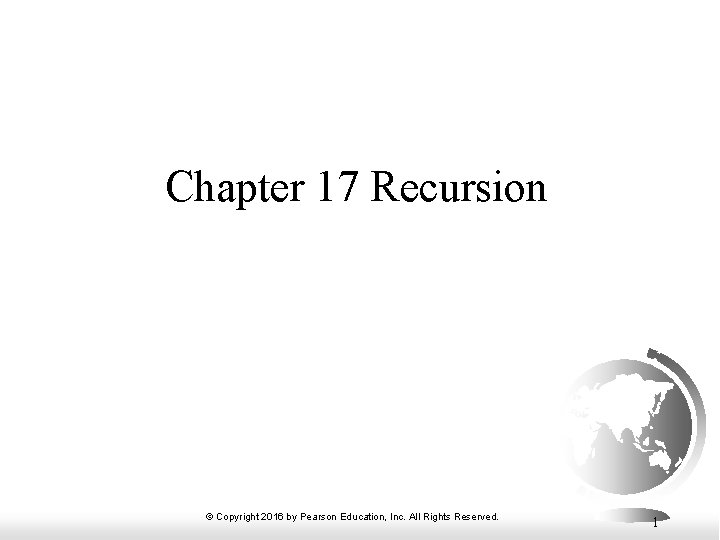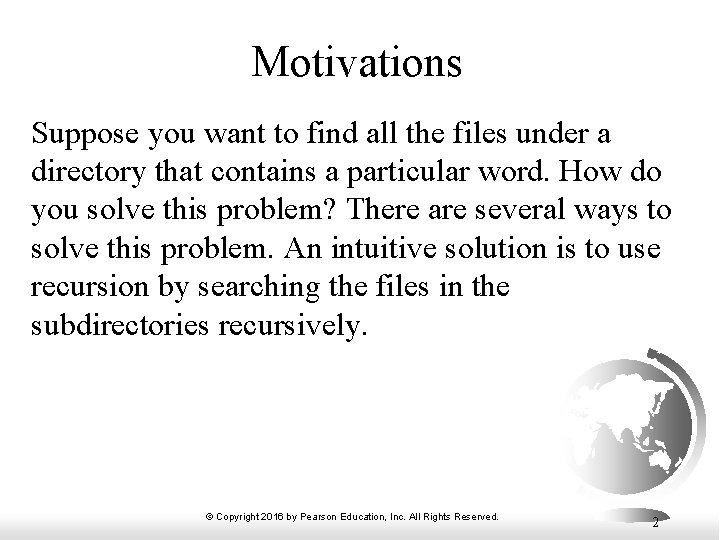Motivations Suppose you want to find all the files under a directory that contains a particular word. How do you solve this problem? There are several ways to solve this problem. An intuitive solution is to use recursion by searching the files in the subdirectories recursively. © Copyright 2016 by Pearson Education, Inc. All Rights Reserved. 2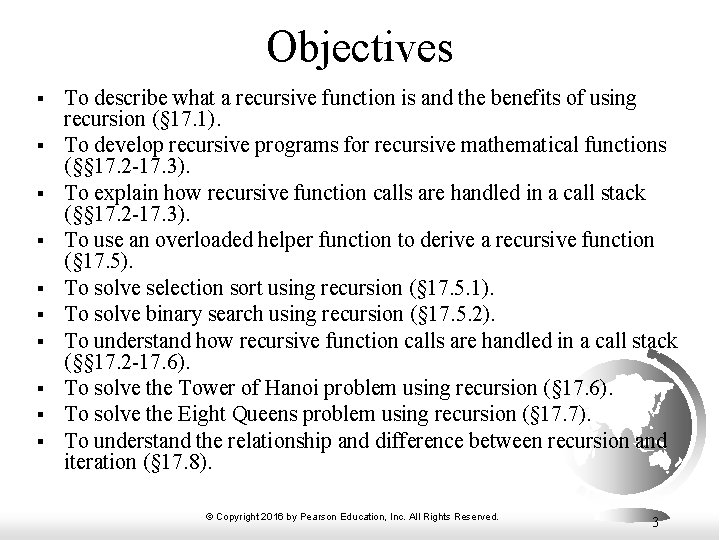Objectives § § § § § To describe what a recursive function is and the benefits of using recursion (§ 17. 1). To develop recursive programs for recursive mathematical functions (§§ 17. 2 -17. 3). To explain how recursive function calls are handled in a call stack (§§ 17. 2 -17. 3). To use an overloaded helper function to derive a recursive function (§ 17. 5). To solve selection sort using recursion (§ 17. 5. 1). To solve binary search using recursion (§ 17. 5. 2). To understand how recursive function calls are handled in a call stack (§§ 17. 2 -17. 6). To solve the Tower of Hanoi problem using recursion (§ 17. 6). To solve the Eight Queens problem using recursion (§ 17. 7). To understand the relationship and difference between recursion and iteration (§ 17. 8). © Copyright 2016 by Pearson Education, Inc. All Rights Reserved. 3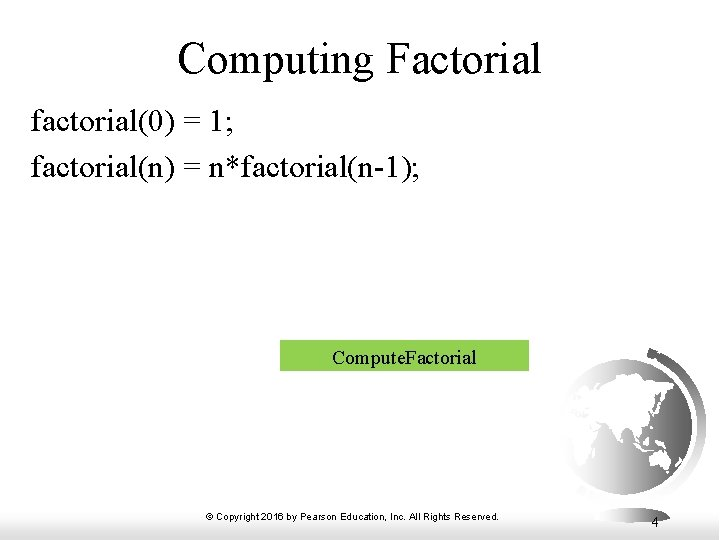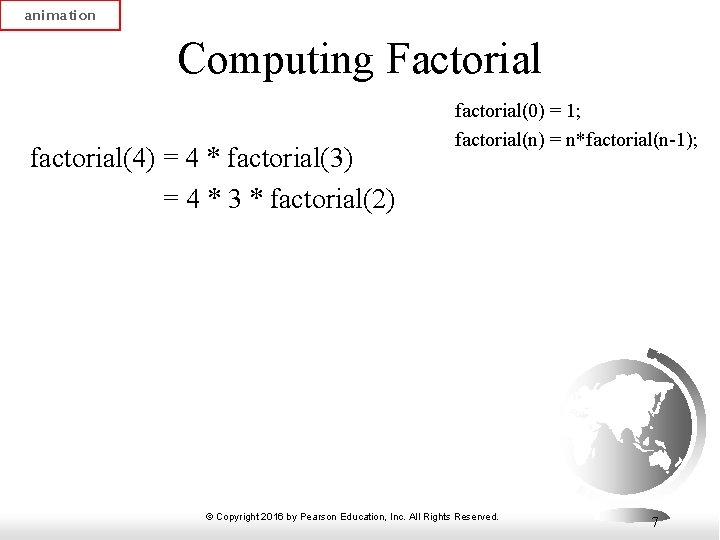animation Computing Factorial factorial(4) = 4 * factorial(3) = 4 * 3 * factorial(2) factorial(0) = 1; factorial(n) = n*factorial(n-1); © Copyright 2016 by Pearson Education, Inc. All Rights Reserved. 7animation Computing Factorial factorial(0) = 1; factorial(n) = n*factorial(n-1); factorial(4) = 4 * factorial(3) = 4 * 3 * factorial(2) = 4 * 3 * (2 * factorial(1)) © Copyright 2016 by Pearson Education, Inc. All Rights Reserved. 8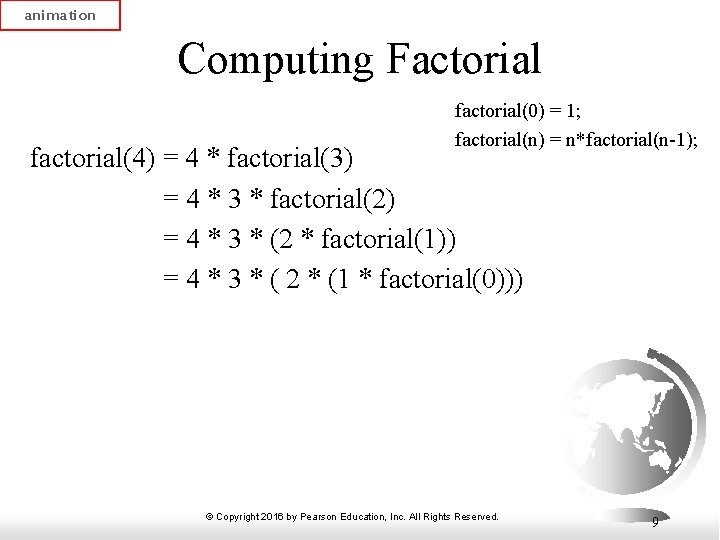animation Computing Factorial factorial(0) = 1; factorial(n) = n*factorial(n-1); factorial(4) = 4 * factorial(3) = 4 * 3 * factorial(2) = 4 * 3 * (2 * factorial(1)) = 4 * 3 * ( 2 * (1 * factorial(0))) © Copyright 2016 by Pearson Education, Inc. All Rights Reserved. 9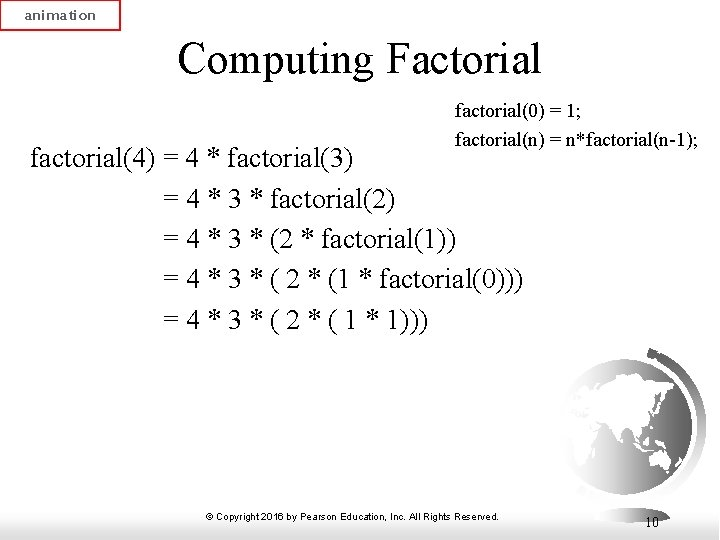animation Computing Factorial factorial(0) = 1; factorial(n) = n*factorial(n-1); factorial(4) = 4 * factorial(3) = 4 * 3 * factorial(2) = 4 * 3 * (2 * factorial(1)) = 4 * 3 * ( 2 * (1 * factorial(0))) = 4 * 3 * ( 2 * ( 1 * 1))) © Copyright 2016 by Pearson Education, Inc. All Rights Reserved. 10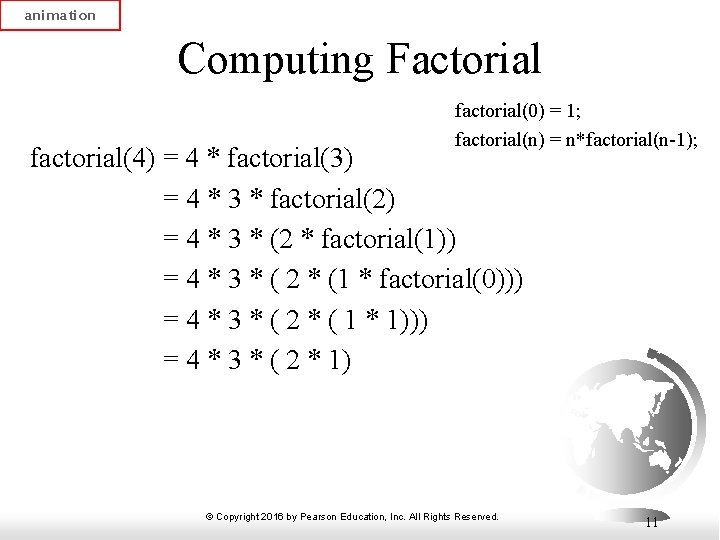animation Computing Factorial factorial(0) = 1; factorial(n) = n*factorial(n-1); factorial(4) = 4 * factorial(3) = 4 * 3 * factorial(2) = 4 * 3 * (2 * factorial(1)) = 4 * 3 * ( 2 * (1 * factorial(0))) = 4 * 3 * ( 2 * ( 1 * 1))) = 4 * 3 * ( 2 * 1) © Copyright 2016 by Pearson Education, Inc. All Rights Reserved. 11animation Computing Factorial factorial(0) = 1; factorial(n) = n*factorial(n-1); factorial(4) = 4 * factorial(3) = 4 * 3 * factorial(2) = 4 * 3 * (2 * factorial(1)) = 4 * 3 * ( 2 * (1 * factorial(0))) = 4 * 3 * ( 2 * ( 1 * 1))) = 4 * 3 * ( 2 * 1) =4*3*2 © Copyright 2016 by Pearson Education, Inc. All Rights Reserved. 12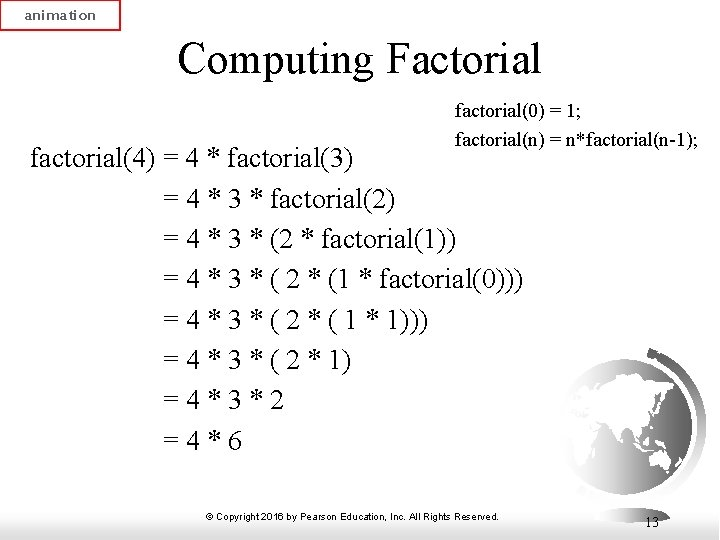animation Computing Factorial factorial(0) = 1; factorial(n) = n*factorial(n-1); factorial(4) = 4 * factorial(3) = 4 * 3 * factorial(2) = 4 * 3 * (2 * factorial(1)) = 4 * 3 * ( 2 * (1 * factorial(0))) = 4 * 3 * ( 2 * ( 1 * 1))) = 4 * 3 * ( 2 * 1) =4*3*2 =4*6 © Copyright 2016 by Pearson Education, Inc. All Rights Reserved. 13animation Computing Factorial factorial(0) = 1; factorial(n) = n*factorial(n-1); factorial(4) = 4 * factorial(3) = 4 * 3 * factorial(2) = 4 * 3 * (2 * factorial(1)) = 4 * 3 * ( 2 * (1 * factorial(0))) = 4 * 3 * ( 2 * ( 1 * 1))) = 4 * 3 * ( 2 * 1) =4*3*2 =4*6 = 24 © Copyright 2016 by Pearson Education, Inc. All Rights Reserved. 14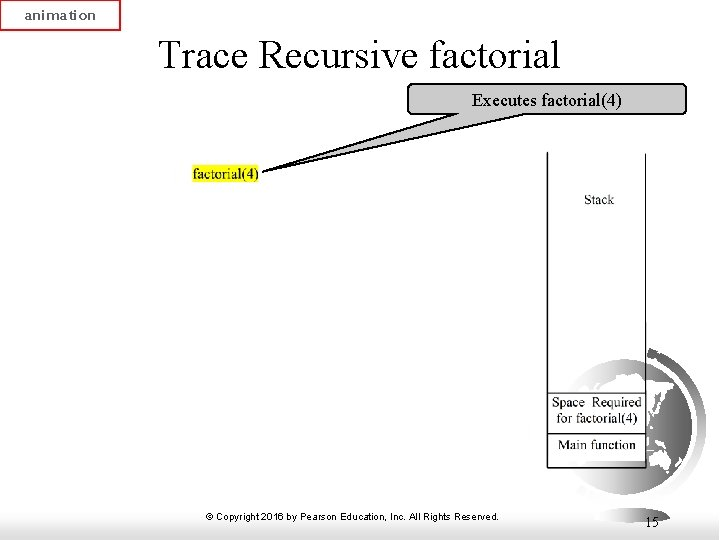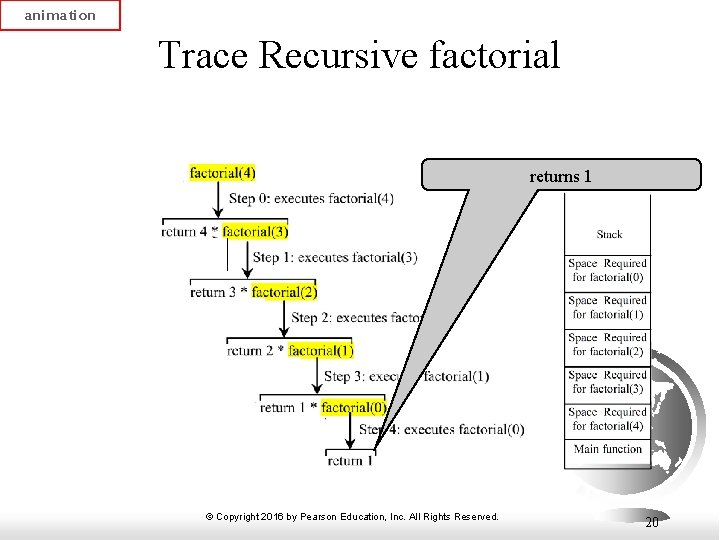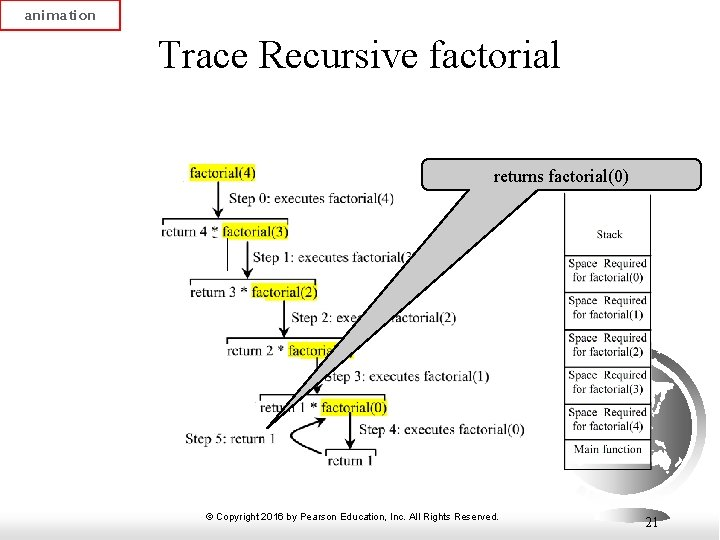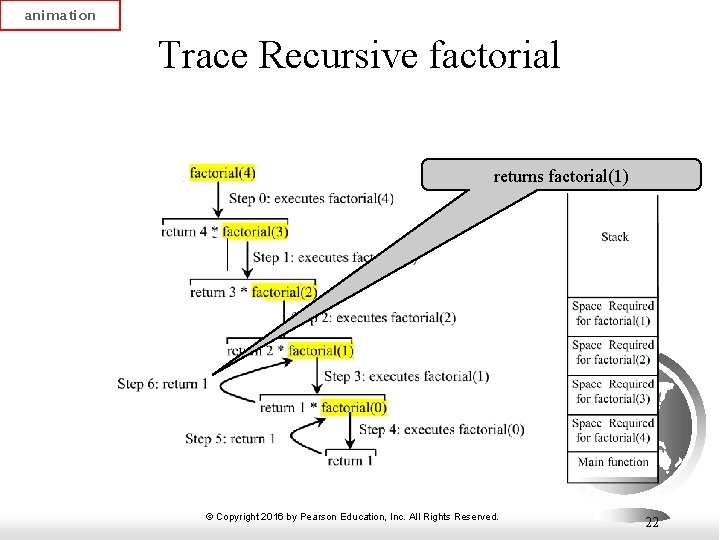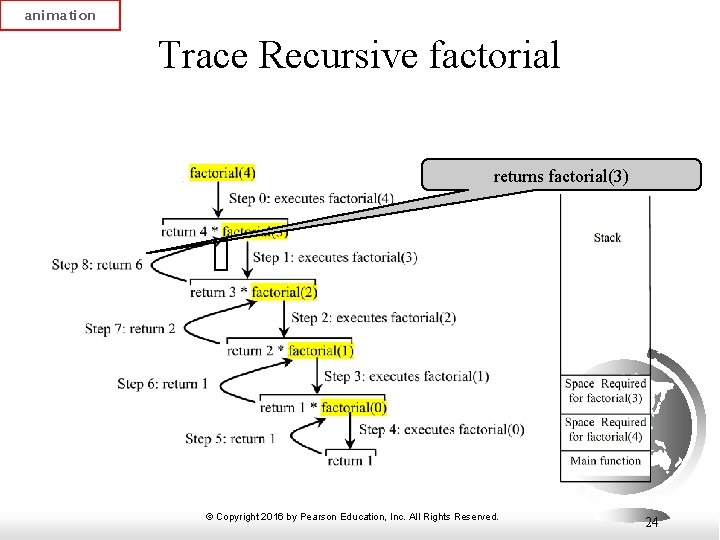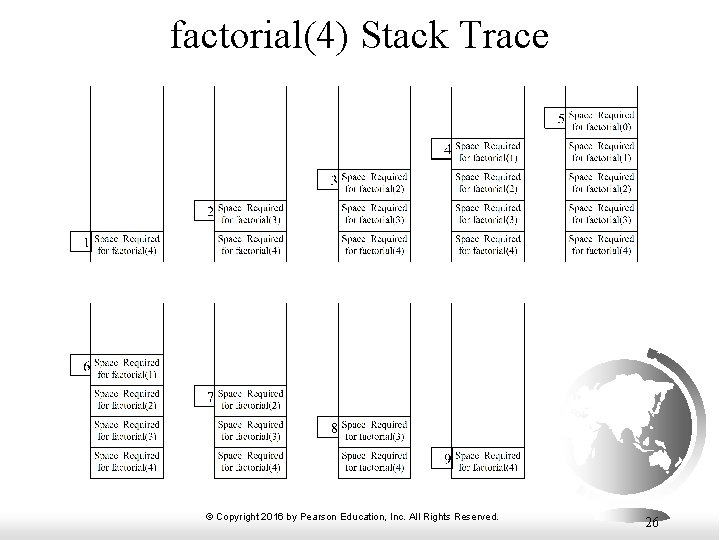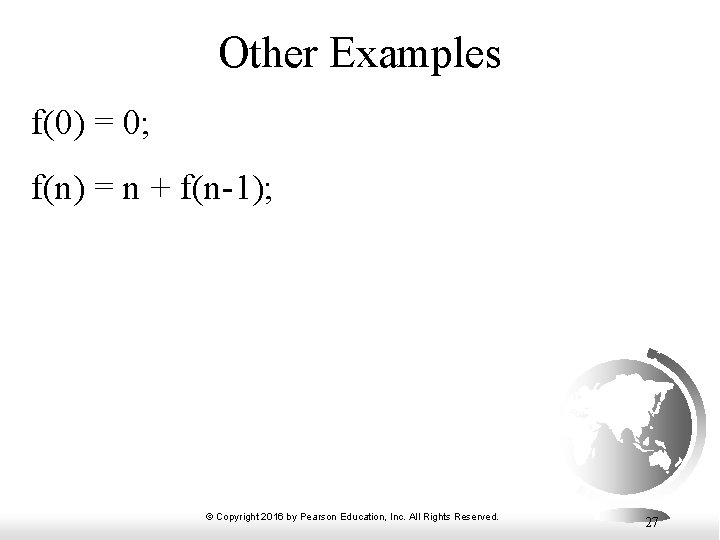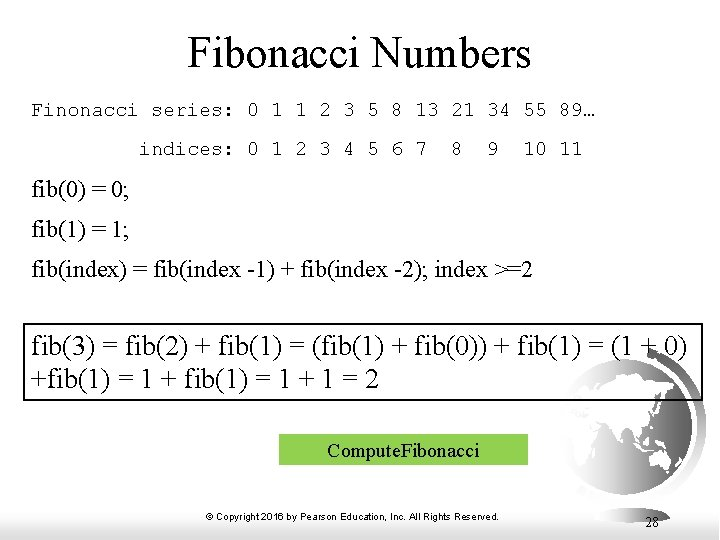Fibonacci Numbers Finonacci series: 0 1 1 2 3 5 8 13 21 34 55 89… indices: 0 1 2 3 4 5 6 7 8 9 10 11 fib(0) = 0; fib(1) = 1; fib(index) = fib(index -1) + fib(index -2); index >=2 fib(3) = fib(2) + fib(1) = (fib(1) + fib(0)) + fib(1) = (1 + 0) +fib(1) = 1 + 1 = 2 Compute. Fibonacci © Copyright 2016 by Pearson Education, Inc. All Rights Reserved. 28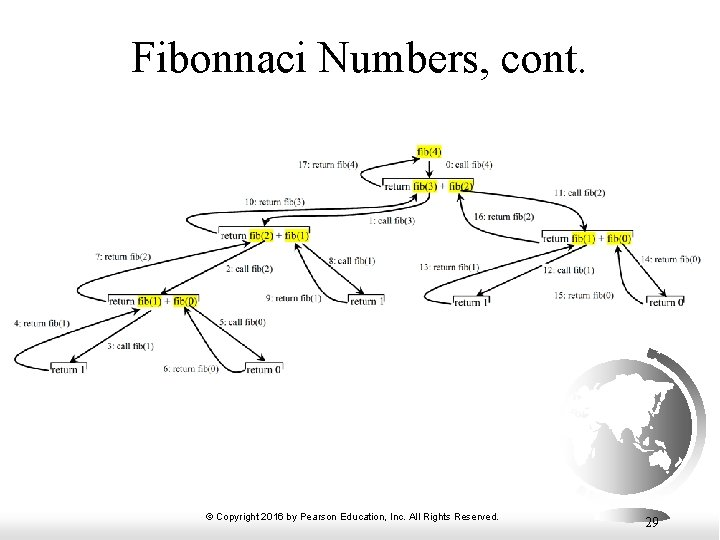Characteristics of Recursion All recursive functions have the following characteristics: – One or more base cases (the simplest case) are used to stop recursion. – Every recursive call reduces the original problem, bringing it increasingly closer to a base case until it becomes that case. In general, to solve a problem using recursion, you break it into subproblems. If a subproblem resembles the original problem, you can apply the same approach to solve the subproblem recursively. This subproblem is almost the same as the original problem in nature with a smaller size. © Copyright 2016 by Pearson Education, Inc. All Rights Reserved. 30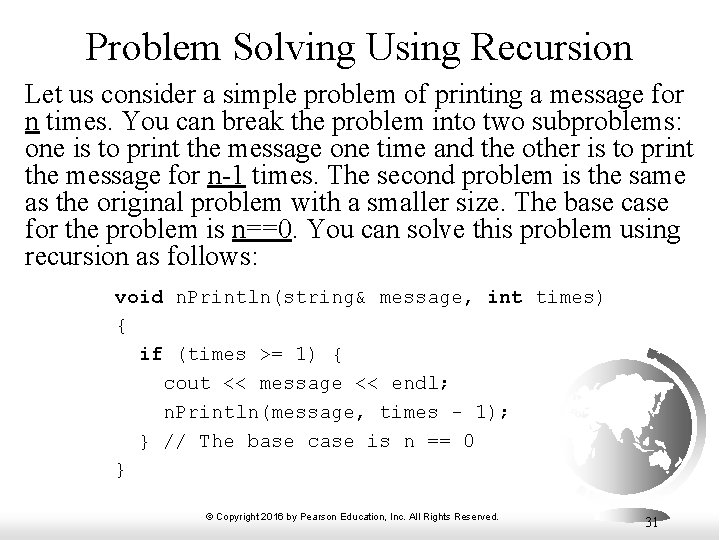Problem Solving Using Recursion Let us consider a simple problem of printing a message for n times. You can break the problem into two subproblems: one is to print the message one time and the other is to print the message for n-1 times. The second problem is the same as the original problem with a smaller size. The base case for the problem is n==0. You can solve this problem using recursion as follows: void n. Println(string& message, int times) { if (times >= 1) { cout << message << endl; n. Println(message, times - 1); } // The base case is n == 0 } © Copyright 2016 by Pearson Education, Inc. All Rights Reserved. 31Think Recursively Many of the problems presented in the early chapters can be solved using recursion if you think recursively. For example, the palindrome problem in Listing 7. 16 can be solved recursively as follows: bool is. Palindrome(const string& s) { if (s. size() <= 1) // Base case return true; else if (s != s[s. size() - 1]) // Base case return false; else return is. Palindrome(s. substr(1, s. size() - 2)); } Recursive. Palindrome © Copyright 2016 by Pearson Education, Inc. All Rights Reserved. 32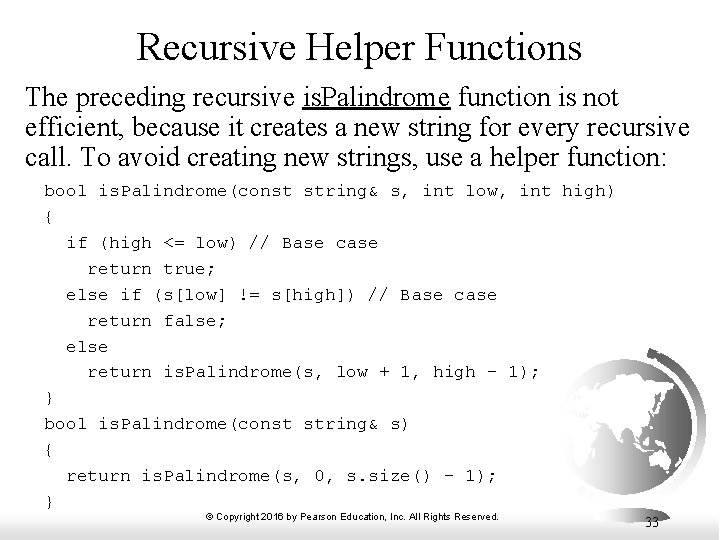Recursive Helper Functions The preceding recursive is. Palindrome function is not efficient, because it creates a new string for every recursive call. To avoid creating new strings, use a helper function: bool is. Palindrome(const string& s, int low, int high) { if (high <= low) // Base case return true; else if (s[low] != s[high]) // Base case return false; else return is. Palindrome(s, low + 1, high - 1); } bool is. Palindrome(const string& s) { return is. Palindrome(s, 0, s. size() - 1); } © Copyright 2016 by Pearson Education, Inc. All Rights Reserved. 33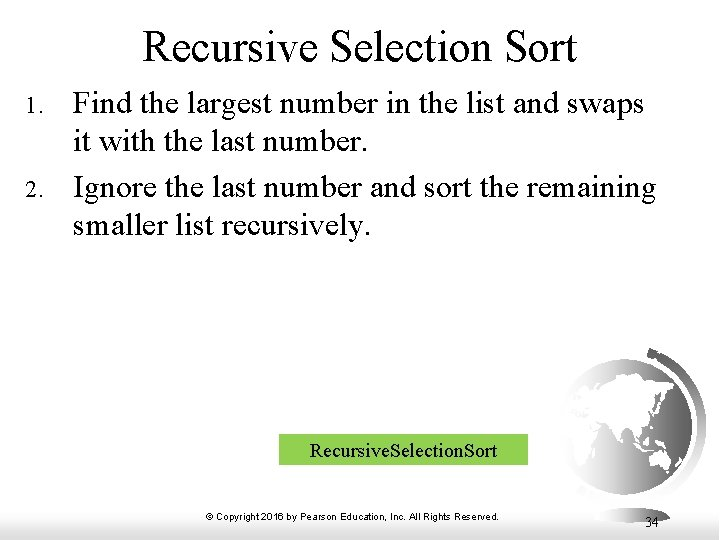Recursive Selection Sort 1. 2. Find the largest number in the list and swaps it with the last number. Ignore the last number and sort the remaining smaller list recursively. Recursive. Selection. Sort © Copyright 2016 by Pearson Education, Inc. All Rights Reserved. 34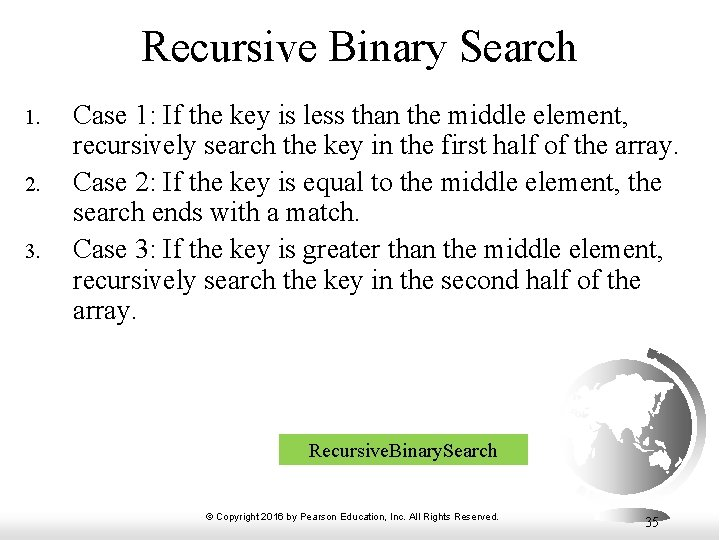Recursive Binary Search 1. 2. 3. Case 1: If the key is less than the middle element, recursively search the key in the first half of the array. Case 2: If the key is equal to the middle element, the search ends with a match. Case 3: If the key is greater than the middle element, recursively search the key in the second half of the array. Recursive. Binary. Search © Copyright 2016 by Pearson Education, Inc. All Rights Reserved. 35Recursive Implementation int binary. Search(const int list[], int key, int low, int high) { if (low > high) // The list has been exhausted without a match return -low - 1; // Return -insertion point - 1 int mid = (low + high) / 2; if (key < list[mid]) return binary. Search(list, key, low, mid - 1); else if (key == list[mid]) return mid; else return binary. Search(list, key, mid + 1, high); } int binary. Search(const int list[], int key, int size) { int low = 0; int high = size - 1; return binary. Search(list, key, low, high); } © Copyright 2016 by Pearson Education, Inc. All Rights Reserved. 36Tower of Hanoi § § There are n disks labeled 1, 2, 3, . . . , n, and three towers labeled A, B, and C. No disk can be on top of a smaller disk at any time. All the disks are initially placed on tower A. Only one disk can be moved at a time, and it must be the top disk on the tower. © Copyright 2016 by Pearson Education, Inc. All Rights Reserved. 37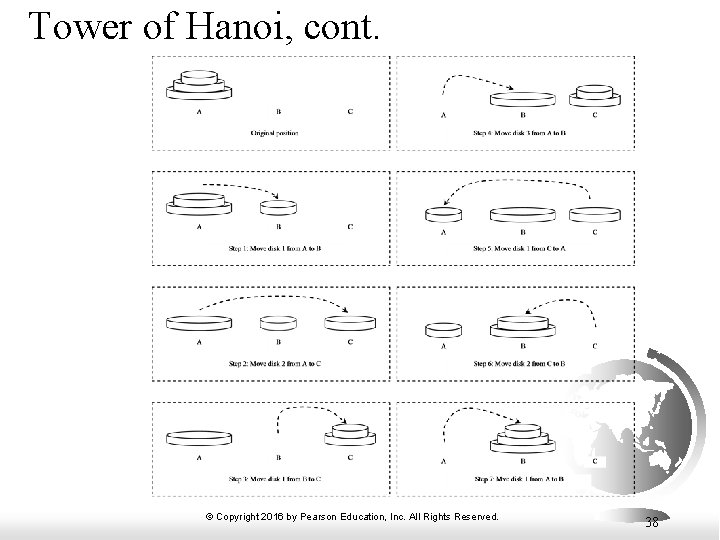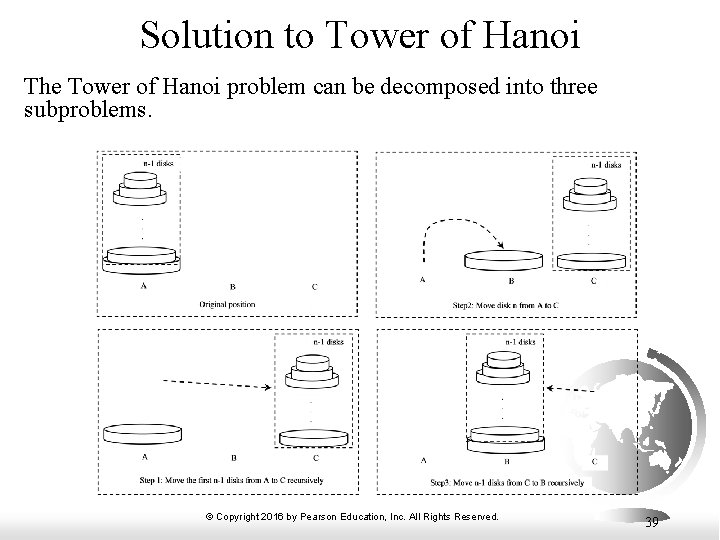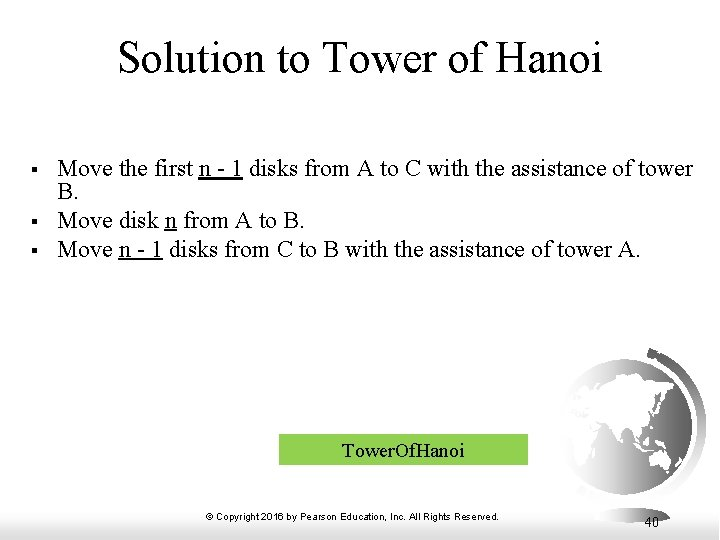Solution to Tower of Hanoi § § § Move the first n - 1 disks from A to C with the assistance of tower B. Move disk n from A to B. Move n - 1 disks from C to B with the assistance of tower A. Tower. Of. Hanoi © Copyright 2016 by Pearson Education, Inc. All Rights Reserved. 40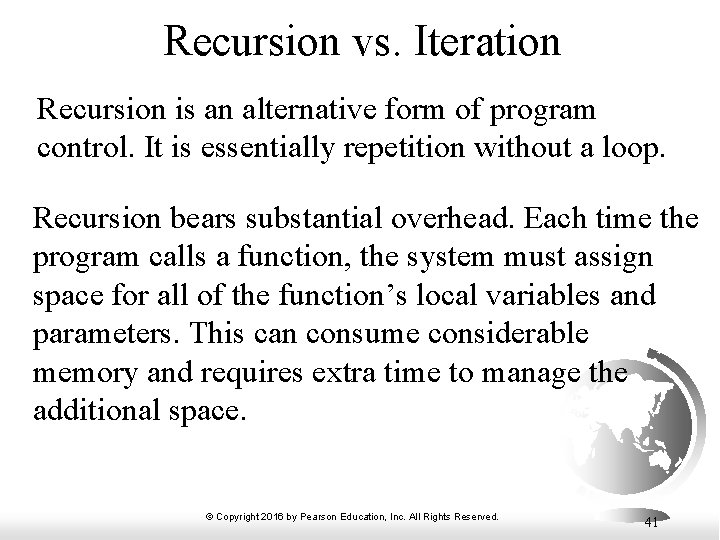Recursion vs. Iteration Recursion is an alternative form of program control. It is essentially repetition without a loop. Recursion bears substantial overhead. Each time the program calls a function, the system must assign space for all of the function’s local variables and parameters. This can consume considerable memory and requires extra time to manage the additional space. © Copyright 2016 by Pearson Education, Inc. All Rights Reserved. 41